# Solving Equations Solving Radical Equations and Inequalities How

• Slides: 11Solving Equations Solving. Radical Equations and. Inequalities • How do we solve radical equations and inequalities? Holt. Mc. Dougal Algebra 2 Holt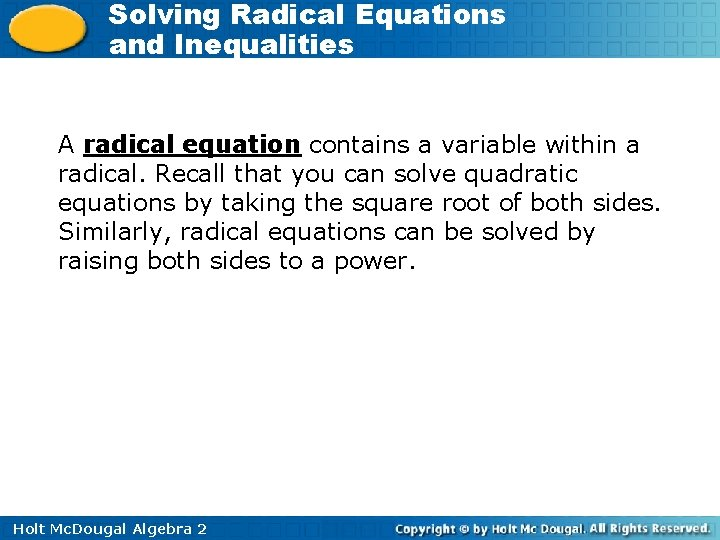Solving Radical Equations and Inequalities A radical equation contains a variable within a radical. Recall that you can solve quadratic equations by taking the square root of both sides. Similarly, radical equations can be solved by raising both sides to a power. Holt Mc. Dougal Algebra 2Solving Radical Equations and Inequalities Remember! For a square root, the index of the radical is 2. Holt Mc. Dougal Algebra 2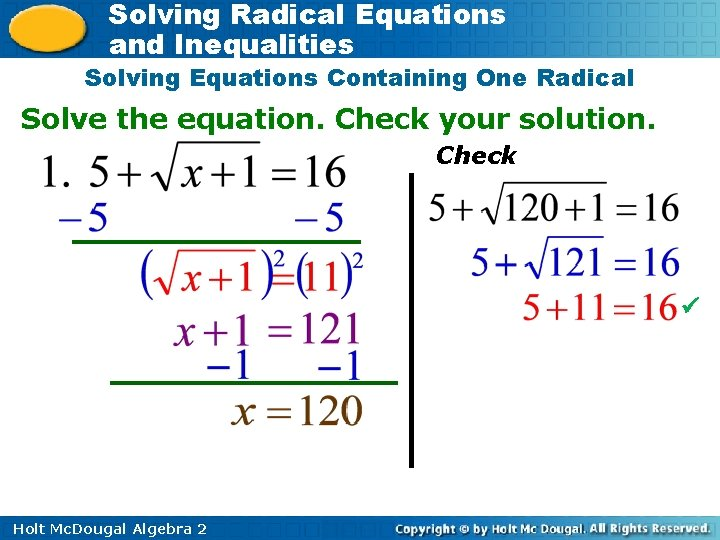Solving Radical Equations and Inequalities Solving Equations Containing One Radical Solve the equation. Check your solution. Check Holt Mc. Dougal Algebra 2Solving Radical Equations and Inequalities Solving Equations Containing One Radical Solve the equation. Check your solution. Check Holt Mc. Dougal Algebra 2Solving Radical Equations and Inequalities Solving Equations Containing One Radical Solve the equation. Check your solution. Check Holt Mc. Dougal Algebra 2Solving Radical Equations and Inequalities Solving Equations Containing One Radical Solve the equation. Check your solution. Check Holt Mc. Dougal Algebra 2Solving Radical Equations and Inequalities Solving Equations Containing Two Radicals Solve the equation. Check your solution. Check Holt Mc. Dougal Algebra 2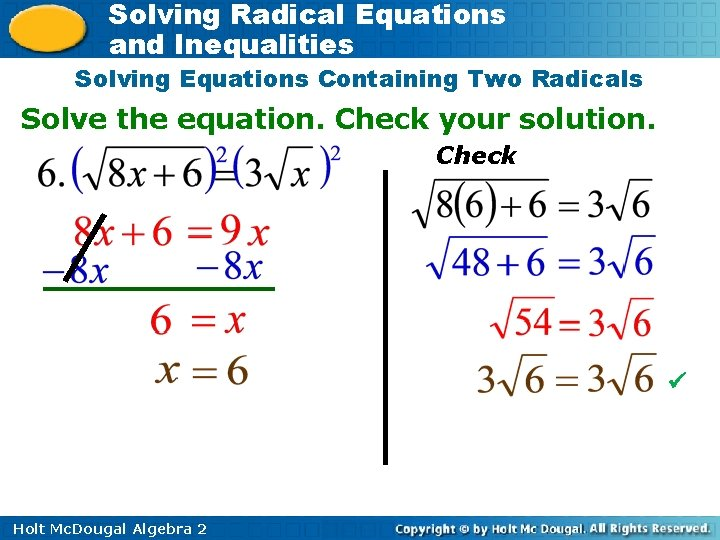Solving Radical Equations and Inequalities Solving Equations Containing Two Radicals Solve the equation. Check your solution. Check Holt Mc. Dougal Algebra 2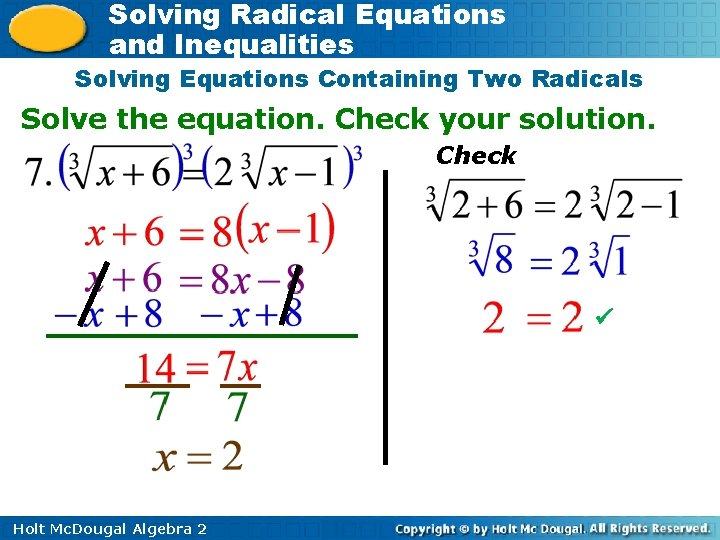Solving Radical Equations and Inequalities Solving Equations Containing Two Radicals Solve the equation. Check your solution. Check Holt Mc. Dougal Algebra 2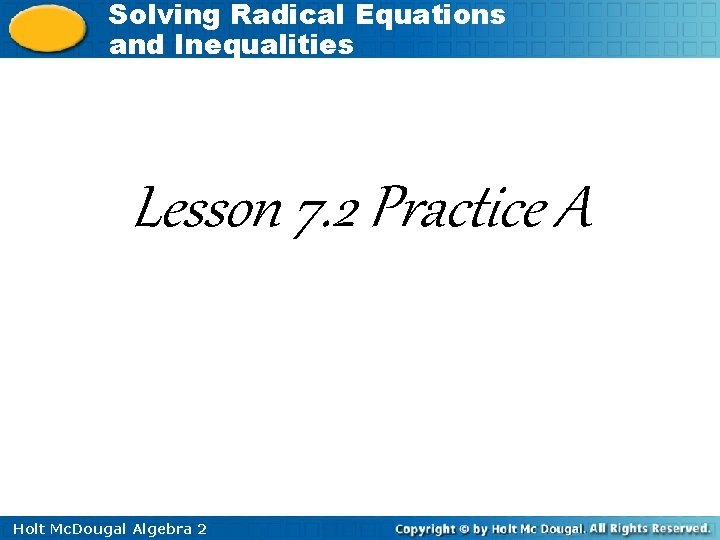Solving Radical Equations and Inequalities Lesson 7. 2 Practice A Holt Mc. Dougal Algebra 2